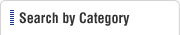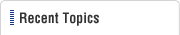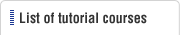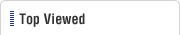HOME  > Plastic Molding Tutorial  > #006 Basic Knowledge on the Mold Clamping Force
Plastic Molding Tutorial

#006 Basic Knowledge on the Mold Clamping Force

Category : Molding Technology
August 7, 2009

When a injection mold is fixed in a molding machine and molten plastic is injected into the interior of the cavity from the injection nozzle, a high filling pressure acts on the inside of the cavity. Since the parting surfaces of the mold try to expand outward due to this pressure, it is necessary to clamp the mold so that it does not open instantaneously.

It is easy to imagine that flash will be generated if the parting surfaces open even very slightly. The force of keeping the mold closed tightly is called the "required mold clamping force". The unit for the required mold clamping force is N (Newtons), or kfg, of tf.

At the time of designing a new mold, it is necessary to obtain by theoretical calculations what is the optimum required mold clamping force that the injection molding machine has to have for the mold to be installed in it. For example, if a required mold clamping force of 100 tf was obtained by calculations, if this mold is installed in an injection molding machine with a 75 tf capacity, the molded product will be full of flash thereby making it impossible to carry out the molding operation. Further, if the mold is installed in a molding machine with a 300 tf capacity, even if the molding operation is possible, since usually the hourly cost of a 300 tf machine is higher than that of a 100 tf machine, the molding operation becomes high in cost.

The required mold clamping force of a mold can be calculated using the following equation.

 F = p×A/1000, where, F: Required mold clamping force (tf), p: pressure inside the cavity (kgf/cm2), and A: total projection area (cm2)

Here, p will have a value in the range of 300 to 500 kgf/cm2. The value of p varies depending on the type of plastic, molded item wall thickness, cavity surface temperature, molding conditions, etc. To be more accurate, it is recommended to incorporate a pressure sensor inside the cavity, and to collect guideline data from actual measured values. Also, A is the total projection area of the cavity and the runner with respect to the parting surface. Therefore, the value of A varies depending on the number of items molded and on the placement of the runner.

##### Example of a Calculation

Consider calculating the required mold clamping force when four molded items are obtained using PBT plastic with 30% glass fibers added.
Let us assume that the assumptions for calculation are that the pressure inside the cavity is P = 300 kgf/cm2, the projection area of one cavity is A1 = 15.3 cm2, and the projection area of the runner is A2 = 5.5 cm2.

F = p×A/ 1000
= 300×(15.3×4+5.5)/1000
= 20.01(tf)

Therefore, an injection molding machine that has a required mold clamping force of about 20 tf is required. Giving some margin, it is considered optimum to select an injection molding machine with a 25 to 30 tf rating.